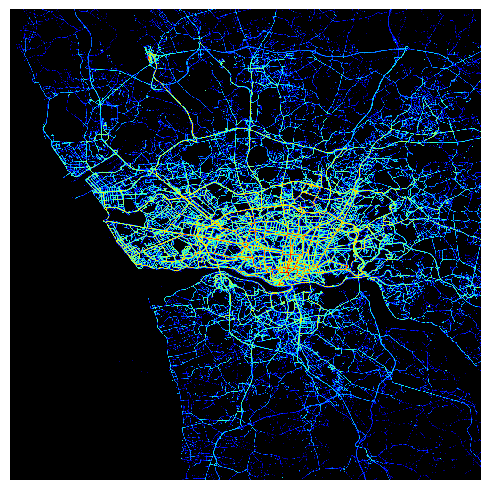# Drawing geographical density maps with Matplotlib

19 December 2016

When dealing with geographical datasets it is sometimes useful to get a visual representation of the data's spatial distribution (or density). Thankfully this is quite simple and efficient to achieve in Python with a Matplotlib histogram. To illustrate this we'll use the dataset provided in Kaggle's ECML/PKDD 15: Taxi Trajectory Prediction competition. This dataset contains millions of GPS coordinates recorded for all taxi trips taken in Porto, Portugal, over a complete year.

First, let's import all the libraries we need:

In :
import copy
import numpy as np
from matplotlib import pyplot as plt
from matplotlib.colors import LogNorm
%matplotlib inline


In :
data = load_data()


(Note: load_data is just a custom function to load data from the CSV files provided for the competition. The code for that function isn't really relevant for the purposes of this blog post, but you may find it on Github if you're interested.)

The POLYLINE_FULL column contains all GPS coordinates — in the format (latitude, longitude) — for each trip. Here's a small sample for the first few trips:

In :
data.train['POLYLINE_FULL'].head()

Out:
553433     [(41.14575, -8.610849), (41.145912, -8.610903)...
164647     [(41.146137, -8.617509), (41.137425, -8.614656...
439067     [(41.146164, -8.617554), (41.145696, -8.617518...
619178     [(41.165019, -8.628219), (41.164767, -8.628111...
1629758    [(41.154444, -8.649792), (41.154372, -8.649783...
Name: POLYLINE_FULL, dtype: object

See a full list of coordinates for a given trip:

In :
print data.train['POLYLINE_FULL'].iloc

[(41.14575, -8.610849), (41.145912, -8.610903), (41.146047, -8.610786), (41.146623, -8.61003), (41.147073, -8.608977), (41.147559, -8.60868), (41.148396, -8.60823), (41.149134, -8.607744), (41.149908, -8.60724), (41.150556, -8.606961), (41.150637, -8.606916), (41.151069, -8.60661), (41.152401, -8.605818), (41.153454, -8.605521), (41.153526, -8.607528), (41.153544, -8.609733), (41.153625, -8.611785), (41.154831, -8.611983), (41.155164, -8.613063), (41.155164, -8.613081), (41.155371, -8.613594), (41.155794, -8.615727), (41.156028, -8.617437), (41.156073, -8.617824), (41.156433, -8.61993), (41.156775, -8.621694), (41.157126, -8.623836), (41.157549, -8.626338), (41.157954, -8.627985), (41.15871, -8.628786), (41.158728, -8.629578), (41.158737, -8.629821), (41.158611, -8.6301), (41.15862, -8.630145), (41.158611, -8.630118), (41.158593, -8.630118)]


Now let's merge all GPS coordinates for all trips in a flat list:

In :
all_coordinates = np.concatenate(data.train['POLYLINE_FULL'].as_matrix())


We now have a list of about 80 millions coordinates:

In :
print all_coordinates.shape
print all_coordinates

(78356174, 2)
[[ 41.14575   -8.610849]
[ 41.145912  -8.610903]
[ 41.146047  -8.610786]
...,
[ 41.156073  -8.627787]
[ 41.156073  -8.627787]
[ 41.156082  -8.627769]]


Here is now the code of our density map function:

In :
def density_map(latitudes, longitudes, center, bins=1000, radius=0.1):
cmap = copy.copy(plt.cm.jet)
cmap.set_bad((0,0,0))  # Fill background with black

# Center the map around the provided center coordinates
histogram_range = [
]

fig = plt.figure(figsize=(5,5))
plt.hist2d(longitudes, latitudes, bins=bins, norm=LogNorm(),
cmap=cmap, range=histogram_range)

# Remove all axes and annotations to keep the map clean and simple
plt.grid('off')
plt.axis('off')
fig.axes.get_xaxis().set_visible(False)
fig.axes.get_yaxis().set_visible(False)
plt.tight_layout()
plt.show()


LogNorm normalizes a given value to the 0-1 range on a logarithmic scale. Note that LogNorm flags 0-value pixels — i.e. areas not covered by the provided GPS coordinates — as "bad" and will color them in white by default. I think the contrasts look better with a dark background, so here we tweak the color map to color those pixels in black instead. Finally, the bins parameter allows you control the contrast of colors for the GPS points themselves.

Now let's draw the density map for all GPS coordinates and center it around Porto:

In :
# Coordinates of Porto's city center
porto = [41.1579, -8.6291]
# Separate the latitude and longitude values from our list of coordinates
latitudes = all_coordinates[:,0]
longitudes = all_coordinates[:,1]
# Render the map
density_map(latitudes, longitudes, center=porto)This rendering process is pretty interesting as it clearly highligthts Porto's busiest roads, the airport in the North West corner, as well as the coast line and the river estuary contours.